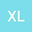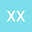A new reproducing kernel method for solving the fractional differential equations
••• xueqin lv,
• Xianjing Xu
xueqin lv
Harbin Normal University

Corresponding Author:xueqinhsd@163.com

Author ProfileXianjing Xu
Harbin Normal University
Author Profile## Abstract

In this paper, we investigate an efficient technique for solving fractional differential equations (FDEs). The proposed technique is based upon Legendre polynomials to construct reproducing kernel spaces, the ε-approximate method is presented in space, and stability and convergence analysis are given by analyzing the condition number of the matrix of the linear system. Finally, comparison with the existing algorithm by the numerical experiments illustrates that efficiency and stability of the proposed method.